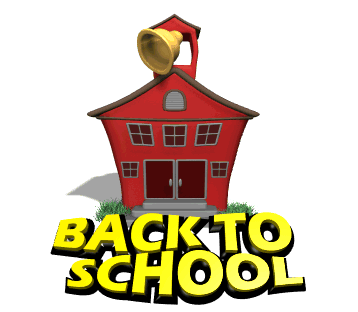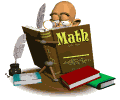## State of the Classroom Flipgrid Video

In my monthly newsletters I provide a peek at upcoming content, common mistakes made in current units, as well as some helpful suggestions about math and organization.  Click on the picture next to each month for that month's newsletter.

September• # Welcome to Pre-Algebra with Mr. Mains!What is Pre-Algebra?

Pre-Algebra is an advanced course for sixth graders which prepares students to enter Algebra I as seventh graders. The focus of the course is building the foundation necessary for success in the study of Algebra. Students will become familiar with the following: the properties of mathematics, the language of Algebra, solving one step and two step equations, adding, subtracting, multiplying and dividing rational numbers and integers, solving inequalities, graphing equations and inequalities, proportions, percents, statistics and graphs, probability, and applying algebra to geometry, measurement, introduction to Trigonometry, and the study of polynomials.

The students work through various activities to come to a deep understanding of the mathematical concepts.  The lessons are structured with a launch activity, an explore activity (guided practice), and then a summary.  The summary section of the lesson is where the students summarize what they have learned and take notes. Traditionally the textbook is where students  go when they do not understand something. However, the binder and the Interactive Study Guide is going to be an even more essential component.

Click the scroll on the left for the course syllabus.